# Inverse logistic function

## Definition

The inverse logistic function or log-odds function is a function from the open interval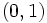$(0,1)$ to all of$\R$ defined as follows: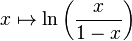$x \mapsto \ln \left(\frac{x}{1 - x}\right)$

The function may be extended to a function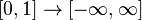$[0,1] \to [-\infty,\infty]$ with the value at 0 defined as$-\infty$ and the value at 1 defined as$\infty$.

### Probabilistic interpretation

Given a probability$p$ (strictly between 0 and 1) the inverse logistic function computes the logarithm of the corresponding odds. Explicitly, the odds corresponding to probability$p$ are:$\frac{p}{1 - p}$

The logarithm of the odds is therefore: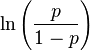$\ln \left(\frac{p}{1 - p}\right)$

## Key data

Item Value
default domain open interval$(0,1)$
range all of$\R$
inverse function logistic function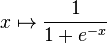$x \mapsto \frac{1}{1 + e^{-x}}$